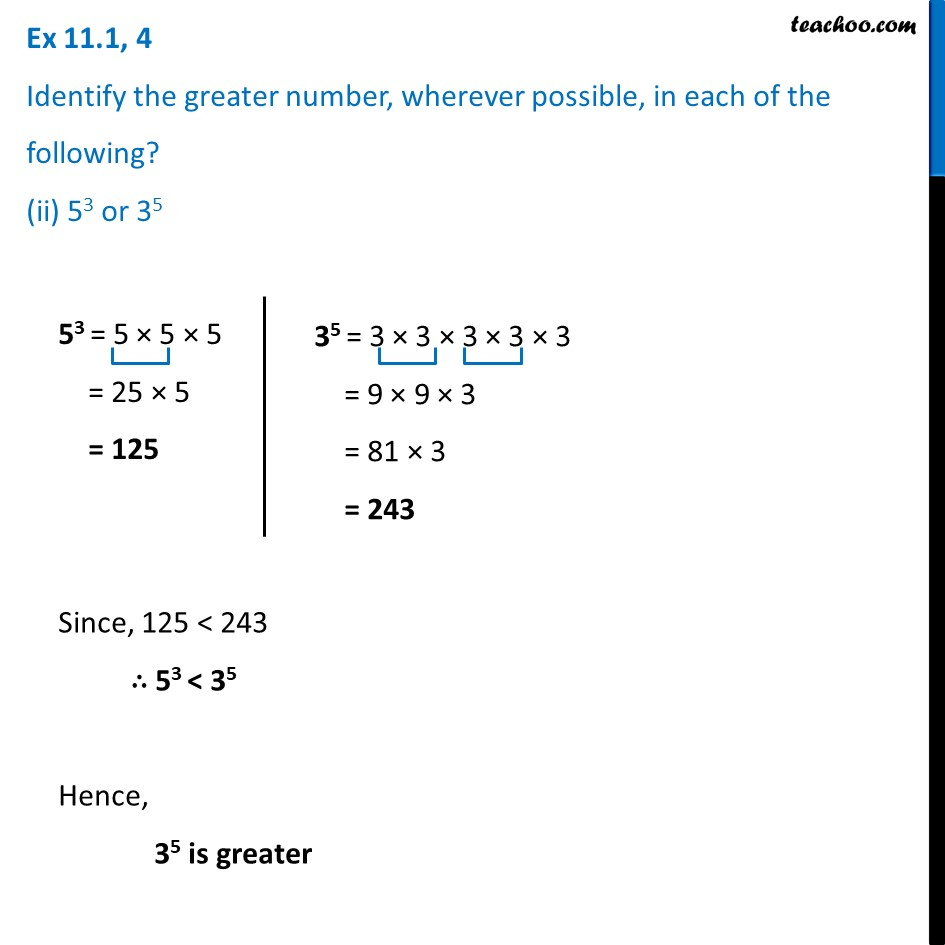Ex 11.1

Chapter 11 Class 7 Exponents and Powers
Serial order wiseLearn in your speed, with individual attention - Teachoo Maths 1-on-1 Class

### Transcript

Ex 11.1, 4 Identify the greater number, wherever possible, in each of the following? (ii) 53 or 35 53 = 5 × 5 × 5 = 25 × 5 = 125 35 = 3 × 3 × 3 × 3 × 3 = 9 × 9 × 3 = 81 × 3 = 243 Since, 125 < 243 ∴ 53 < 35 Hence, 35 is greater